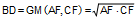# Problem 1105: Triangle, Incircle, Excircle, Circle, Cevian, Tangent, Congruence, Geometric Mean. Level: High School, SAT Prep, College Geometry

< PREVIOUS PROBLEM  |  NEXT PROBLEM >

 In a triangle ABC (see the figure below) the incircle I is tangent to BC, AC, and AB at T1, T2, and T3. The excircle E corresponding to BC is tangent to AC, BC, and AB at F, G, and H, respectively. D is a point on AC so that the incircles of triangles ABD and BDC are congruent. Prove that BD is the geometric mean of AF and CF, that is,.Home | Search | Geometry | Problems | All Problems | Open Problems | Visual Index | 1101-1110 | Triangle | Circle | Incircle | Excircle | Tangent Line | Congruence | Geometric Mean | Email | Post a comment or solutionLast updated: Apr 1, 2015, by Antonio Gutierrez